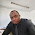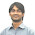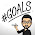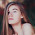Welcome! Dear Traders,you are reading my forex trading experiences. Forex trading is a very profitable and very risky business opportunity. If you are a beginner, calm down,have a cup of coffee, and convince yourself that you need to study hard to win in forex trading. Obviously, the task is not easy as the statistics claim that only 5% traders win in forex trading. If you are determined, serious,and hard working, you can surely be included in the group of winners.

### HOW TO TRADE BULLISH CYPHER HARMONIC PATTERN

yourFXguide-According to investopedia.com "Harmonic price patterns take geometric price patterns to the next level by using Fibonacci numbers to define precise turning points. Unlike other trading methods, Harmonic trading attempts to predict future movements. "

The harmonic pattern explained along this post is "Bullish Cypher Pattern". This pattern is an XABCD pattern. Bullish Cypher Harmonic Chart Pattern suggests an upcoming bullish movement in the price . In the first part of this post, we will learn the way to find a Bullish Cypher Pattern on the practical price chart, and in the second part we will learn the way to trade a Bullish Cypher Pattern.

How to find/draw Bullish Cypher Harmonic Chart Pattern on price chart?

The first step to find a Bullish Cypher harmonic chart pattern is to find the X and A points of the pattern. The X and A points of the pattern is found at the bottom and top of  a strong bullish trend respectively. In the second step, we will draw a fibonacci retracement tool from the X point to the A point of the pattern to find the B point of the pattern. The B point should be 38.2%-61.8% fibonacci retracement of XA. We can find the B point of the pattern mathematically without applying the fibonacci retracement tool. The formulas to find the B point mathematically are as follows:

(a) Price at 38.2% of XA = Price at A-(Price at A-Price at X) X 0.382
(b) Price at 61.8% of XA = Price at A-(Price at A-Price at X) X 0.618

The B point should be lied on the range between 38.2%-61.8% fibonacci retracement.

In third step, we are to draw a fibonacci retracement tool from A point to B point of the pattern to find the C point of the pattern. The C point should be in the range of 113%-141.4% Fibonacci retracement. To find this range mathematically we can apply the following formulas:

(a) Price at 113% of AB = Price at B+(Price at A-Price at B) X 1.13.
(b) Price at 141.4% of AB= Price at B+(Price at A-Price at B) X 1.414.

In fourth step, we will draw a fibonacci retracement tool from B point to C point to find the D point of the pattern. The D point should be in the range of 127.2%-200% fibonacci retracement level. To find this range mathematically, we can apply the following formulas:

(a) Price at 127.2% of BC = Price at C-(Price at C-Price at B) X 1.272.
(b) Price at 200% of BC= Price at C-(Price at C-Price at B) X 2.00.

D point is confirmed by two conditions because it is the most crucial point of the pattern. In fifth step we will draw a fibonacci retracement tool from point X to point C to reconfirm point D. The D point should be at the 78.6% fibonacci retracement of XC. The mathematical formula to find the price level at D point is as follows.

(a) Price at 78.6% of XC= Price at C-(Price at C-Price at X) X 0.786.

In the last and final step, all points are added by straight lines to find the Bullish Cypher Pattern. This step is not necessary to be performed by the traders. They just need to find all five points of the pattern with the fibonacci ratios or retracement levels.

How to trade Bullish Cypher Harmonic Chart Pattern?

The commonly practiced trading strategy to trade a Bullish Cypher harmonic chart pattern is placing a buy order when the D point of the pattern is confirmed. The stop loss for the order placed at D point is generally placed little lower than the lower fibonacci retracement level.

Profit target for the order placed at D point can be placed at different fibonacci retracement levels of CD. To do so, a fibonacci retracement tool is drawn from C point to D point. The most widely placed profit target are at the 38.2% and 50% fibonacci retracement of CD, but can be placed at larger retracement level depending on the market situation.

The profit target can also be placed at the vertical lines through B point and A point.

Some smart traders also like to place order at B and C points. In my later posts, I will explain the way to trade these points.

Dear Traders,If you have any question regarding this post, you can drop it into the comment section below. I generally respond to your comments within 24 hours.

#### 8 comments :

1.Which time frame is the best to plot the Cypher wave hourly or30 mins

2.There are no specific time frames to apply bullish cypher pattern, you can apply it on any charts greater than 30m time frames.

Thanks

3.I learned thad D = 0.786 of XC. Why are you teaching this like that?

1.I am doing the same thing dear !!!

2.D = 78.6 of XA

4.Forex mini trading is a way to trade using much less capital than 99% of people realize is even possible. Find out the details from someone who knows... indicator mt4

5.It is simpler to use the fibonacci expansion tool and using 100% is the same result.

6.The unconditional guarantee is one of the features that we really like as it gives confidence to individuals who wish to try it check out the review here.review here

Drop a comment...